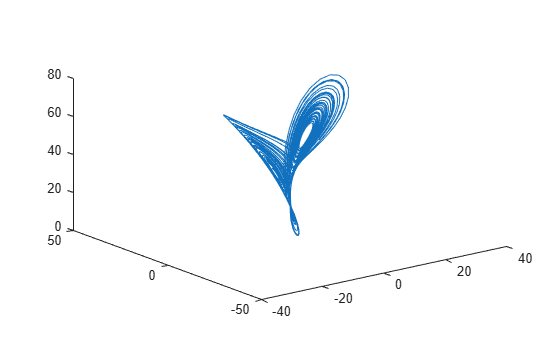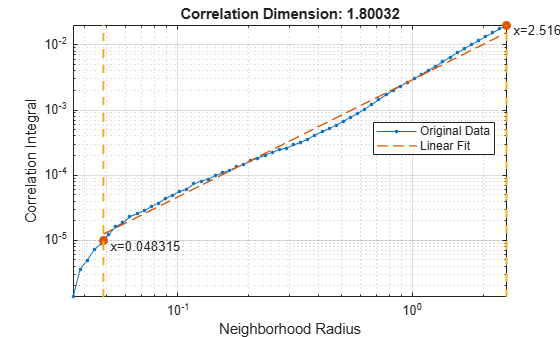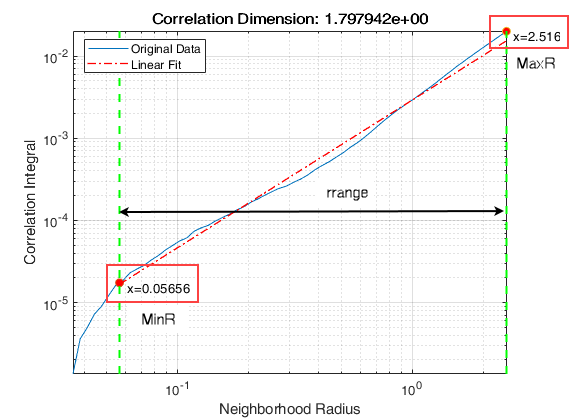correlationDimension

Measure of chaotic signal complexity

Description

example

corDim = correlationDimension(X) estimates the correlation dimension of the uniformly sampled time-domain signal X. Correlation dimension is the measure of dimensionality of the space occupied by a set of random points. corDim is estimated as the slope of the correlation integral versus the range of radius of similarity. Use correlationDimension as a characteristic measure to distinguish between deterministic chaos and random noise, to detect potential faults.

example

corDim = correlationDimension(X,lag) estimates the correlation dimension of the uniformly sampled time-domain signal X for the time delay lag.

example

corDim = correlationDimension(X,[],dim) estimates the correlation dimension of the uniformly sampled time-domain signal X for the embedding dimension dim.

example

corDim = correlationDimension(X,lag,dim) estimates the correlation dimension of the uniformly sampled time-domain signal X for the time delay lag and embedding dimension dim.

example

[corDim,rRange,corInt] = correlationDimension(___) additionally estimates the range of radius of similarity and correlation integral of the uniformly sampled time-domain signal X. Correlation integral is the mean probability that the states of a system are close at two different time intervals, which reflects self-similarity.

example

___ = correlationDimension(___,Name,Value) estimates the correlation dimension with additional options specified by one or more Name,Value pair arguments.

example

correlationDimension(___) with no output arguments creates a correlation integral versus neighborhood radius plot.

Examples

collapse all

In this example, consider a Lorenz Attractor describing a unique set of chaotic solutions.

Load the data set and visualize the Lorenz Attractor in 3D.

plot3(data(:,1),data(:,2),data(:,3));For this example, use only x-direction data of the Lorenz Attractor. Since lag is unknown, estimate the delay using phaseSpaceReconstruction. Set 'Dimension' to 3 since the Lorenz Attractor is a three-dimensional system. The dim and lag parameters are required to create the correlation integral versus the neighborhood radius plot.

xdata = data(:,1);
dim = 3;
[~,lag] = phaseSpaceReconstruction(xdata,[],dim)
lag = 10

Create the correlation integral versus neighborhood radius plot for the Lorenz Attractor, using the lag value obtained in the previous step. Set an appropriate value for 'NumPoints' to determine a good resolution for the neighborhood radius.

Np = 100;
correlationDimension(xdata,lag,dim,'NumPoints',Np);The first dashed, vertical green line (on the left) indicates the value of MinRadius, while the second vertical green line (on the right), represents MaxRadius. The dashed red line indicates the linear fit line for the correlation integral versus neighborhood radius data, within the computed range of radius.

To compute correlation dimension, you first need to determine the MinRadius and MaxRadius values needed for accurate estimation.

In the plot, drag the two dashed, vertical green lines to 'best fit' the linear fit line to the original data line to obtain the range of radius.Note the new values of MinRadius and MaxRadius after dragging the two vertical lines for an appropriate fit.

Find the correlation dimension of the Lorenz Attractor, using the new MinRadius and MaxRadius values obtained in the previous step.

MinR = 0.05656;
MaxR = 2.516;
corDim = 1.7490

The value of correlation dimension is directly proportional to the level of chaos in the system, that is, a higher value of corDim represents a high level of chaotic complexity in the system.

Input Arguments

collapse all

Uniformly sampled time-domain signal, specified as a vector, array, or timetable. If X has multiple columns, correlationDimension computes the correlation dimension by treating X as a multivariate signal.

If X is specified as a row vector, correlationDimension treats it as a univariate signal.

Embedding dimension, specified as a scalar or vector. dim is equivalent to the 'Dimension' name-value pair.

Time delay, specified as a scalar or vector. lag is equivalent to the 'Lag' name-value pair.

Name-Value Arguments

Specify optional comma-separated pairs of Name,Value arguments. Name is the argument name and Value is the corresponding value. Name must appear inside quotes. You can specify several name and value pair arguments in any order as Name1,Value1,...,NameN,ValueN.

Example: ...,'Dimension',3

Embedding dimension, specified as the comma-separated pair consisting of 'Dimension' and a scalar or vector. When Dimension is scalar, every column in X is reconstructed using Dimension. When Dimension is a vector having same length as the number of columns in X, the reconstruction dimension for column i is Dimension(i).

Specify Dimension based on the dimension of your system, that is, the number of states. For more information on embedding dimension, see phaseSpaceReconstruction.

Delay in phase space reconstruction, specified as the comma-separated pair consisting of 'Lag' and either a scalar or vector. When Lag is scalar, every column in X is reconstructed using Lag. When Lag is a vector having same length as the number of columns in X, the reconstruction delay for column i is Lag(i).

If the delay is too small, random noise is introduced in the data. In contrast, if the lag is too large, the reconstructed dynamics does not represent the true dynamics of the time series. For more information on estimating optimal delay, see phaseSpaceReconstruction.

Minimum radius of similarity, specified as the comma-separated pair consisting of 'MinRadius' and a scalar. Find the optimal value of MinRadius by adjusting the linear fit of the correlation dimension plot.

Maximum radius of similarity, specified as the comma-separated pair consisting of 'MaxRadius' and a scalar. Find the optimal value of MaxRadius by adjusting the linear fit of the correlation dimension plot.

Number of points for computation, specified as the comma-separated pair consisting of 'NumPoints' and a positive scalar integer. NumPoints is the number of points between MinRadius and MaxRadius. Choose an appropriate value for NumPoints based on the resolution required for rRange.

NumPoints only accepts values greater than 1, and the default value is 10.

Output Arguments

collapse all

Correlation dimension, returned as a scalar. corDim is a measure of chaotic signal complexity in multidimensional phase space and is the slope of the correlation integral versus the range of radius of similarity. corDim is used in fault detection as a characteristic measure to distinguish between deterministic chaos and random noise.

Radius of similarity, returned as an array. rRange is the difference between MaxRadius and MinRadius split into an equal number of points defined by NumPoints.

Correlation integral, returned as an array. corInt is the mean probability that the states at two different times are close, which reflects self-similarity. NumPoints defines the length of corInt array.

Algorithms

Correlation dimension is computed in the following way,

1. The correlationDimension function first generates a delayed reconstruction Y1:N with embedding dimension m, and lag τ.

2. The software then calculates the number of with-in range points, at point i, given by,

${N}_{i}\left(R\right)=\sum _{i=1,i\ne k}^{N}1\left(‖{Y}_{i}-{Y}_{k}‖

where 1 is the indicator function, and R is the radius of similarity, given by, R = exp(linspace(log(rmin), log(rmax), N)). Here, rmin is MinRadius, rmax is MaxRadius, and N is NumPoints.

3. The correlation dimension corDim is the slope of C(R) vs. R where, the correlation integral C(R) is defined as,

$C\left(R\right)=\frac{2}{N\left(N-1\right)}\sum _{i=1}^{N}{N}_{i}\left(R\right)$

 Caesarendra, Wahyu & Kosasih, P & Tieu, Kiet & Moodie, Craig. "An application of nonlinear feature extraction-A case study for low speed slewing bearing condition monitoring and prognosis." IEEE/ASME International Conference on Advanced Intelligent Mechatronics: Mechatronics for Human Wellbeing, AIM 2013.1713-1718. 10.1109/AIM.2013.6584344.

 Theiler, James. "Efficient algorithm for estimating the correlation dimension from a set of discrete points". American Physical Society. Physical Review A 1987/11/1. Volume 36. Issue 9. Pages 44-56.